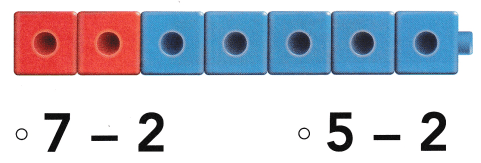# Texas Go Math Kindergarten Lesson 10.5 Answer Key Decompose 6 and 7

Refer to our Texas Go Math Kindergarten Answer Key Pdf to score good marks in the exams. Test yourself by practicing the problems from Texas Go Math Kindergarten Lesson 10.5 Answer Key Decompose 6 and 7.

## Texas Go Math Kindergarten Lesson 10.5 Answer Key Decompose 6 and 7

Essential Question
How do you take away numbers from 6 and 7?

Explore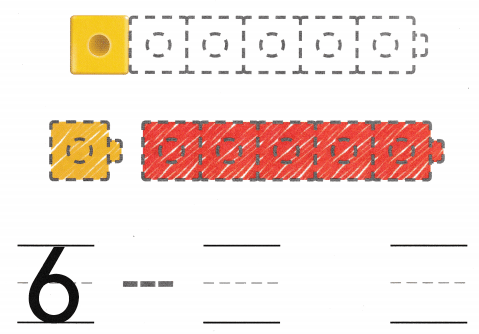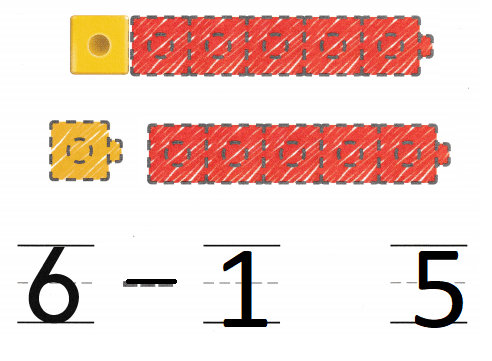Explanation:
One cube is yellow and the rest are red
so, 6 – 1 = 5
out of 6 one cube is yellow the remaining are red.

Directions
Model a six-cube train. One cube is yellow and the rest are red. Take apart the train to model the set token apart.
Draw and color the cube trains. Trace the symbol and write the numbers to show 6 taken apart.

Share and Show

Question 1.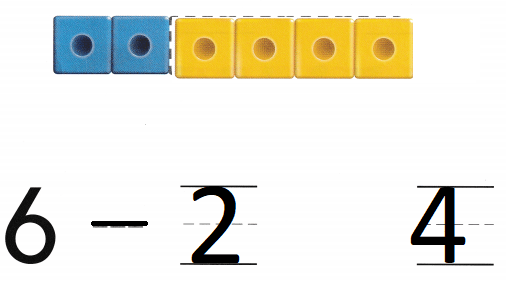Explanation:
Number of cubes are 6
number of blue cubes are 2
6 – 2 = 4
so, there are 4 yellow cubes.

Question 2.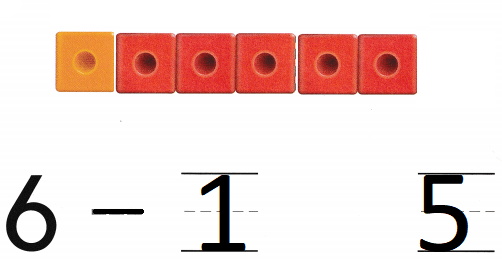Explanation:
Number of cubes are 6
number of orange cubes are 1
6 – 1 = 5
so, there are 5 red cubes.

Directions
Model a six-cube train. One cube is yellow and the rest are red. Take apart the train to model the set taken apart. Draw and color the cube trains. Trace the symbol and write the numbers to show 6 taken apart.

Question 3.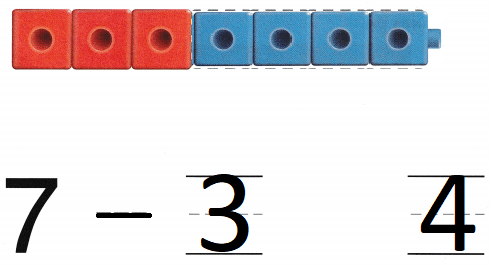Explanation:
Number of cubes are 7
number of red cubes are 3
7 – 3 = 4
so, there are 4 blue cubes.

Question 4.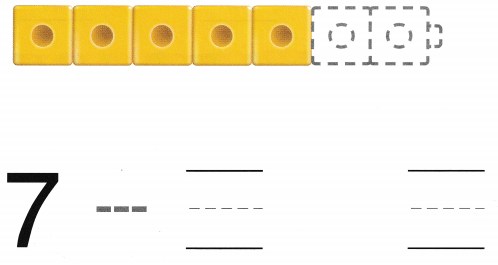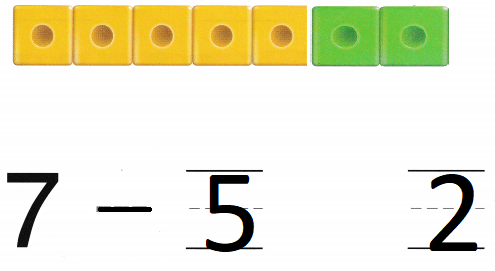Explanation:
Number of cubes are 7
number of yellow cubes are  5
7 – 5 = 2
so, there are 2 green cubes.

Directions
3. Model a seven-cube train. Three cubes are red and the rest are blue. Take apart the train to model the set taken apart. Draw and color the cube trains, Trace the symbol and write the numbers to show 7 taken apart. 4. Model a seven-cube train, Five cubes are yellow and the rest are green. Take apart the train to model the set taken apart. Draw and color the cube trains. Trace the symbol and write the numbers to show 7 taken apart.

Home Activity

• Show your child six or seven small objects. Take apart the set of objects. Have him or her tell a word problem to match the subtraction.

Problem Solving

Question 5.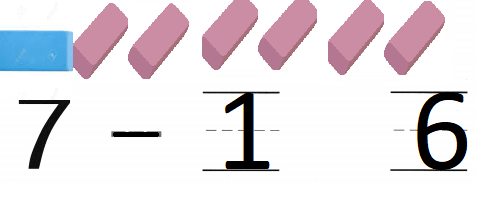Explanation:
Juli has seven erasers. One eraser is blue.
The rest of the erasers are pink.
7 – 1 = 6
so, 6 erasers are pink.

Question 6.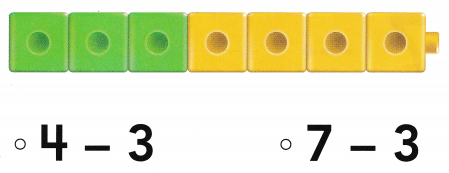Explanation:
Number of cubes are 7
number of green cubes are 3
7 – 3 = 4
so, there are 4 yellow cubes.

Directions
5. Juli has seven erasers. One eraser is blue. The rest of the erasers are pink. How many erasers are pink? Draw the erasers. Trace the symbol and write the numbers. 6. Choose the correct answer. Which does the cube train show?

### Texas Go Math Kindergarten Lesson 10.5 Homework and Practice

Question 1.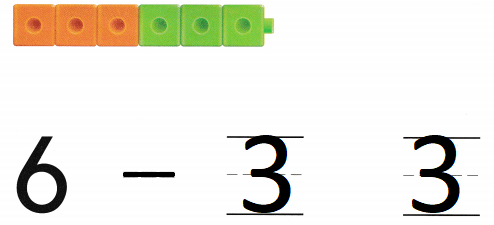Explanation:
Number of cubes are 6
number of orange cubes are 3
and number of green cubes are 3
6 – 3 = 3

Question 2.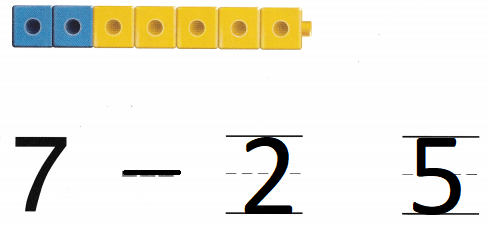Explanation:
Number of cubes are 7
number of yellow cubes are  5
7 – 5 = 2
so, there are 2 blue cubes.

Directions
1. Look at the six-cube train. Three cubes are orange. The rest are green. Take apart the train by drawing to model the set taken apart. Color the cube trains. Trace and write to show 6 taken apart. 2. Look at the seven-cube train. Two cubes are blue The rest are yellow. Take apart the train by drawing to model the set taken apart. Color the cube trains. Trace and write to show 7 taken apart.

Texas Test Prep

Lesson Check

Question 3.Explanation:
Total number of cubes are 6
out of them 4 are orange and the remaining are 2
6 – 4 = 2

Question 4.